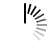# Looping Techniques in Java

1 Answer

The different looping techniques in Java using various loops are given as follows.

## for loop

The for loop contains the initialization, condition and the increment/decrement in a single line. So it is shorter and more concise as compared to the while or do while loops.

A program that demonstrates the for loop in Java is given as follows −

## Example

Live Demo

```public class Example {
public static void main(String args[]) {
System.out.println("Using for loop...");
for (int i = 1; i <= 10; i++)
System.out.print(i + " ");
}
}
```

## Output

```Using for loop...
1 2 3 4 5 6 7 8 9 10
```

## while loop

The while loop contains a condition and the code in the loop body is executed based on that condition. When the condition becomes false, the program control exits the loop.

A program that demonstrates the while loop in Java is given as follows −

## Example

Live Demo

```public class Example {
public static void main(String args[]) {
int i = 1;
System.out.println("Using while loop...");
while(i <= 10) {
System.out.print(i + " ");
i++;
}
}
}
```

## Output

```Using while loop...
1 2 3 4 5 6 7 8 9 10
```

## do while loop

The do while loop is similar to the while loop except it is an exit controlled loop. This means that the condition is at the end of the loop and is checked after executing the loop body each time.

A program that demonstrates the do while loop in Java is given as follows −

## Example

Live Demo

```public class Example {
public static void main(String args[]) {
int i = 1;
System.out.println("Using do while loop...");
do {
System.out.print(i + " ");
i++;
}while(i <= 10);
}
}
```

## Output

```Using do while loop...
1 2 3 4 5 6 7 8 9 10
```

Advertisements

We use cookies to provide and improve our services. By using our site, you consent to our Cookies Policy.